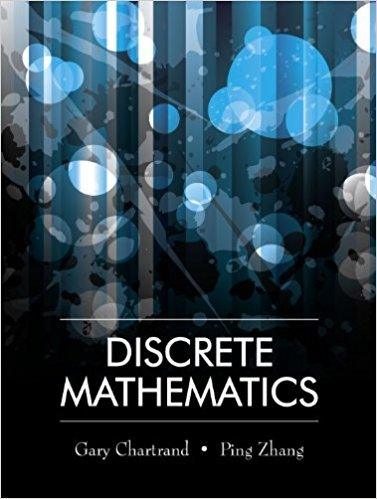×
×

# An investor intends to buy shares of stock in 3 companies, chosen from a list of 12ISBN: 9781577667308 385

## Solution for problem 11 Chapter 8.5

Discrete Mathematics | 1st Edition

• Textbook Solutions
• 2901 Step-by-step solutions solved by professors and subject experts
• Get 24/7 help from StudySoup virtual teaching assistantsDiscrete Mathematics | 1st Edition

4 5 1 423 Reviews
20
0
Problem 11

An investor intends to buy shares of stock in 3 companies, chosen from a list of 12 companies. How many different investment options are there if (a) equal amounts are invested in each company? (b) equal amounts are invested in exactly two companies? (c) different amounts are invested in each company?

Step-by-Step Solution:
Step 1 of 3

L26 - 4 Derivatives and the Shape of a Graph f > 0 f < 0 f > 0 ▯▯ f < 0 ex. Find all relative extrema...

Step 2 of 3

Step 3 of 3

##### ISBN: 9781577667308

Discrete Mathematics was written by and is associated to the ISBN: 9781577667308. The answer to “An investor intends to buy shares of stock in 3 companies, chosen from a list of 12 companies. How many different investment options are there if (a) equal amounts are invested in each company? (b) equal amounts are invested in exactly two companies? (c) different amounts are invested in each company?” is broken down into a number of easy to follow steps, and 51 words. The full step-by-step solution to problem: 11 from chapter: 8.5 was answered by , our top Math solution expert on 03/13/18, 07:11PM. Since the solution to 11 from 8.5 chapter was answered, more than 214 students have viewed the full step-by-step answer. This textbook survival guide was created for the textbook: Discrete Mathematics, edition: 1. This full solution covers the following key subjects: . This expansive textbook survival guide covers 79 chapters, and 1714 solutions.

Unlock Textbook Solution